Courses

# NCERT Solutions(Part - 1) - Data Handling Class 7 Notes | EduRev

## Class 7 : NCERT Solutions(Part - 1) - Data Handling Class 7 Notes | EduRev

The document NCERT Solutions(Part - 1) - Data Handling Class 7 Notes | EduRev is a part of the Class 7 Course Mathematics (Maths) Class 7.
All you need of Class 7 at this link: Class 7

Exercise 3.1
Ques 1: Find the range of heights of any ten students of your class.
Ans:

 S. No. Name of students Height (in feet) 1. Gunjan 4.2 2. Aditi 4.5 3. Nikhil 5 4. Akhil 5.1 5. Riya 5.2 6. Akshat 5.3 7. Abhishek 5.1 8. Mayank 4.7 9. Rahul 4.9 10. Ayush 4.5

Range = Highest height – Lowest height
= 5.3 – 4.2
= 1.1 feet

Ques 2: Organize the following marks in a class assessment, in a tabular form:
4, 6, 7, 5, 3, 5, 4, 5, 2, 6, 2, 5, 1, 9, 6, 5, 8, 4, 6, 7.
(i) Which number is the highest?
(ii) Which number of the lowest?
(iii) What is the range of the numbers?
(iv) Find the arithmetic mean
Ans:

 S. No. Marks Tally marks Frequency (No. of students) 1. 1 I 1 2. 2 II 2 3. 3 I 1 4. 4 III 3 5. 5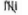5 6. 6 IIII 4 7. 7 II 2 8. 8 I 1 9. 9 I 1

(i) The highest number is 9.
(ii) The lowest number is 1.
(iii) The range of the data is 9 - 1 = 8
(iv) Arithmetic mean
=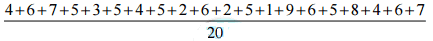= 100/20 = 5

Ques 3: Find the mean of the first five whole numbers.
Ans: The first five whole numbers are 0, 1, 2, 3 and 4.
Therefore,
Mean of first five whole numbers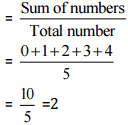Thus, the mean of first five whole numbers is 5.

Ques 4: A cricketer scores the following runs in eight innings: 58, 76, 40, 35, 46, 45, 0, 100 Find the mean score.
Ans: Number of innings = 8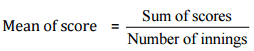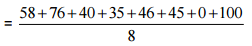= 400/8 = 50
Thus, the mean score is 50.

Ques 5: Following table shows the points of each player scored in four games:

 Player Game 1 Game 2 Game 3 Game 4 A 14 16 10 10 B 0 8 6 4 C 8 11 Did not play 13

(i) Find the mean to determine A’s average number of points scored per game.
(ii) To find the mean number of points per game for C, would you divide the total points by 3 or 47? Why?
(iii) B played in all the four games. How would you find the mean?
(iv) Who is the best performer?
Ans:
(i)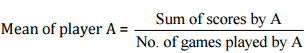(ii) We should divide the total points by 3 because player C played only three games.
(iii) Player B played in all the four games.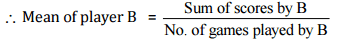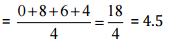(iv) To find the best performer, we should know the mean of all players.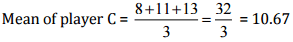Therefore, on comparing means of all players, player A is the best performer.

Ques 6: The marks (out of 100) obtained by a group of students in a science test are 85, 76, 90, 85, 39, 48, 56, 95, 81 and 75.
Find the:
(i) The highest and the lowest marks obtained by the students.
(ii) Range of the marks obtained.
(iii) Mean marks obtained by the group.
Ans: (i) Highest marks obtained by the student = 95
Lowest marks obtained by the student = 39
(ii) Range of marks = Highest marks – Lowest marks
= 95 – 39
= 56
(iii)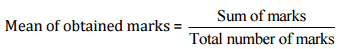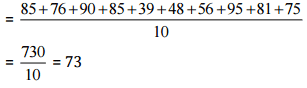Thus, the mean marks obtained by the group of students is 73.

Ques 7: The enrolment in a school during six consecutive years was as follows: 1555, 1670, 1750, 2013, 2540, 2820 Find the mean enrolment of the school for this period.
Ans: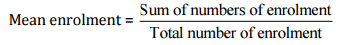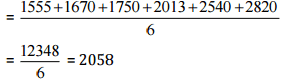Thus, the mean enrolment of the school is 2,058.

Ques 8: The rainfall (in mm) in a city on 7 days of a certain week was recorded as follows:

 Day Mon Tue Wed Thur Fri Sat Sun Rainfall (in mm) 0.0 12.2 2.1 0.0 20.5 5.5 1.0

(i) Find the range of the rainfall in the above data.
(ii) Find the mean rainfall for the week.
(iii) On how many days was the rainfall less than the mean rainfall?
Ans: (i) The range of the rainfall = Highest rainfall – Lowest rainfall
= 20.5 – 0.0 = 20.5 mm
(ii)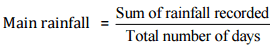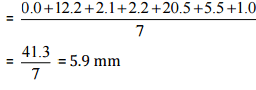(iii) 5 days. i.e., Monday, Wednesday, Thursday, Saturday and Sunday rainfalls were less than the mean rainfall.

Ques 9: The height of 10 girls were measured in cm and the results are as follows: 135, 150, 139, 128, 151, 132, 146, 149, 143, 141
(i) What is the height of the tallest girl?
(ii) What is the height of the shortest girl?
(iii) What is the range of data?
(iv) What is the mean height of the girls?
(v) How many girls have heights more than the mean height?
Ans: (i) The height of the tallest girl = 151 cm
(ii) The height of the shortest girl = 128 cm
(iii) The range of the data = Highest height – Lowest height
= 151 – 128 = 23 cm
(iv)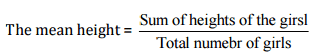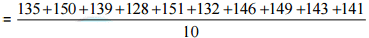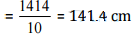(v) Five girls, i.e., 150, 151, 146, 149, 143 have heights (in cm) more than the mean height.

Exercise 3.2
Ques 1: The scores in mathematics test (out of 25) of students is as follows:
19, 25, 23, 20, 9, 20, 15, 10, 5, 16, 25, 20, 24, 12, 20
Find the mode and median of this data. Are they same?
Ans: Arranging the given data in ascending order,
5, 9, 10, 12, 15, 16, 19, 20, 20, 20, 20, 23, 24, 25, 25
Mode is the observation occurred the highest number of times.
Therefore, Mode = 20
Median is the middle observation = 20
Yes, Mode and Median are same of given observation

Ques 2: The runs scored in a cricket match by 11 players is as follows:
6, 15, 120, 50, 100, 80, 10, 15, 8, 10, 15
Find the mean, mode and median of this data. Are the three same?
Ans: Arranging the given data in ascending order, 6, 8, 10, 10, 15, 15, 15, 50, 80, 100, 120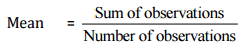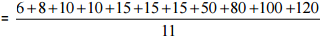= 429/11 = 39
Mode is the observation occurred the highest number of times = 15
Median is the middle observation = 15
Therefore, Mode and Median is 15. No, the mean, median and mode are not same.

Ques 3: The weight (in kg) of 15 students of a class are:
38, 42, 35, 37, 45, 50, 32, 43, 43, 40, 36, 38, 43, 38, 47
(i) Find the mode and median of this data.
(ii) Is there more than one mode?
Ans: Arranging the given data in ascending order,
32, 35, 36, 37, 38, 38, 38, 40, 42, 43, 43, 43, 45, 47, 50
(i) Mode is the observation occurred the highest number of times.
Therefore, Mode = 38 and 43
Median is the middle observation = 40
(ii) Yes, there are 2 modes.

Ques 4: Find the mode and median of the data:
13, 16, 12, 14, 19, 12, 14, 13, 14.
Ans: Arranging the given data in ascending order,
12, 12, 13, 13, 14, 14, 14, 16, 19
Mode is the observation occurred the highest number of times = 14
Median is the middle observation = 14

Ques 5: Tell whether the statement is true or false:
(i) The mode is always one of the numbers in a data.
(ii) The mean is one of the numbers in a data.
(iii) The median is always one of the numbers in a data.
(iv) The data 6, 4, 3, 8, 9, 12, 13, 9 has mean 9.
Ans: (i) True
(ii) False
(iii) True
(iv) False

Offer running on EduRev: Apply code STAYHOME200 to get INR 200 off on our premium plan EduRev Infinity!

## Mathematics (Maths) Class 7

165 videos|222 docs|45 tests

,

,

,

,

,

,

,

,

,

,

,

,

,

,

,

,

,

,

,

,

,

;